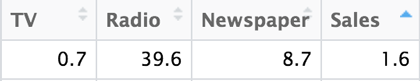# Lesson 13: Combination of Variables

## Lesson 13: Combination of Variables

### Objective:

Students will learn that we can make better predictions by including more variables. Then they will wrestle with how the information should be combined.

### Materials:

1. Advertising Plots Part 2 handout (LMR_4.13_Advertising Plots 2) from Lesson 12

### Essential Concepts:

Essential Concepts:

If multiple predictors are associated with the response variable, a better predictive model will be produced, as measured by the mean absolute error.

### Lesson:

1. Display the plots and statements from the previous day:1. Combining multiple variables (e.g., money spent on TV and Newspaper ads, TV and Radio ads, TV, Radio, and Newspaper ads, etc.) into one model will lead to worse predictions because the variables that make poor predictions will contaminate those that make good predictions.

2. Ask the students to share out their opinions in an Active Debate (see Unit 2 Lesson 6 as an example).

3. Next, inform teams that they will have 2 minutes to come up with as many combinations of ads (variables) as they can think of (e.g., TV + Newspaper ads, TV+ Radio ads, TV + Radio + Newspaper ads, etc.)

4. After 2 minutes, list all the different combinations by conducting a Whip Around and eliciting a combination from each team.

5. By a show of hands, ask students to select which combination or single model will be the best predictor for the number of items sold by the retailer.

6. Then inform students that we will determine which of the statements is true by comparing the mean absolute error (MAE) of single models (like the ones we showed in the previous lesson) vs. combined models. But first, use the line of best fit for the combined variables:

Note: The function that produced the line of best fit using RStudio was

lm(Sales ~ TV + Radio + Newspaper, data= retail)

1. Use this equation to predict the amount of sales for the same market they circled in the previous lesson. Students’ calculation should yield the predicted value in (b), below.

Note: Remind students that they need to substitute the values as they appear in the x-axis of the plots without converting to thousands of dollars. For example, the circled market spent about 10 thousand dollars on newspaper ads, so students should substitute 10 instead of the expanded value in the equation.2. Does the predicted value (10.407)) seem like a plausible number of sales? Why? It is not a plausible number of sales because the prediction is too high. The prediction says the retailer will sell about 104,070 units, when the actual sales were about 16,000 units. Although the model did not make a very good prediction for this market, it is not surprising because as LMR_4.13 displays, that market did not fit the overall pattern in any of the scatterplots.

7. Reveal that RStudio calculated the mean absolute error for different combinations plus the single models, and the results are displayed on the table below. This means that, for example, when using the TV model to predict number of items sold, our predictions will typically be off by about 2.337808 (in 10,000s) of units or 23,378 units. Then ask students:

Model Mean Absolute Error
TV 2.337808
Newspaper 4.538444
TV-Newspaper 2.344971
1. Which model is the best predictor of number of items sold? Answer: The TV-Radio model is the best predictor of number of items sold because it had the least amount of error, on average. When using the TV-Radio model to predict number of items sold, our predictions will typically be off by 11,609 units.

2. Which model was the least reliable in predicting the number of items sold? Answer: The Newspaper model is the least reliable predictor of number of items sold because it had the most amount of error, on average. When using the Newspaper model to predict number of items sold, our predictions will typically be off by 45,384 units.

3. What else do you notice about the models? Answer: It appears that combining the variables into one model is much better than any of the single-variable models.

8. Inform the students that, in the next lab, they will find out how to create the line of best fit for models that include many variables.

### Class Scribes:

One team of students will give a brief talk to discuss what they think the 3 most important topics of the day were.

### Homework & Next Day

Ask students to think of a reason or reasons about why it would not be a good idea to make a scatterplot for models that include more than 3 predictor variables? The answer is mainly because humans are limited to seeing things in 3 dimensions. For example, the model that combines all of the variables together is a 4 dimensional model. What does that look like?

LAB 4E: This Model is Big Enough for All of Us

Complete Lab 4E prior to Practicum.